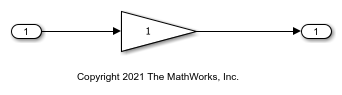# Reuse Parameter Data from External Code in the Generated Code

This example shows how to generate code that imports a parameter value from your external code.

### Create External Code Files

Suppose your external code defines a vector parameter `myGains` with three elements. Save the definition in your current folder in a file `ex_vector_import_src.c`.

```#include "ex_vector_import_decs.h" my_int8 myGains = { 2, 4, 6 }; ```

Save the declaration in your current folder in a file called `ex_vector_import_decs.h`.

```#include "ex_vector_import_cust_types.h" extern my_int8 myGains; ```

Save the data type definition `my_int8` in your current folder in a file called `ex_vector_import_cust_types.h`.

```typedef signed char my_int8; ```

### Import Parameter Value for Simulation

In your current folder, right-click the file `ex_vector_import_src.c` and select Import Data.

In the Import dialog box, set Output Type to `Numeric Matrix`.

Set the name of the generated MATLAB variable to `tempVar`.

Select only the parameter values (`2`, `4`, and `6`) to import.Import the data by clicking the green check mark. The MATLAB variable `tempVar` appears in the base workspace.

Alternatively, use the command prompt to manually create `tempVar`.

```tempVar = [2;4;6]; ```

At the command prompt, create a `Simulink.Parameter` object that represents `myGains`.

```myGains = Simulink.Parameter(tempVar); ```

### Create and Configure Model

Create the model `ex_vector_import`.

```open_system('ex_vector_import') ```Open the Embedded Coder app.

On the Modeling tab, click Model Data Editor.

In the Model Data Editor, inspect the Parameters tab.

Use the Value column to set the value of the Gain parameter in the Gain block to `myGains`.

Click the Show/refresh additional information button. The data table now contains a row that represents the parameter object, `myGains`.

Use the Data Type column to set the data type of `myGains` to `my_int8`.

For the other row (which represents the Gain parameter of the Gain block), set Data Type to `Inherit: Inherit from 'Gain'`. With this setting, the Gain parameter inherits the data type `my_int8` from `myGains`.

Set the Change view drop-down list to `Code`.

Set these properties for `myGains`:

• Storage Class to `ImportFromFile`

• Header File to `ex_vector_import_decs.h`

Alternatively, use these commands at the command prompt to configure the object and the block:

```set_param('ex_vector_import/Gain','Gain','myGains',... 'ParamDataTypeStr','Inherit: Inherit from ''Gain''') myGains.DataType = 'my_int8'; myGains.CoderInfo.StorageClass = 'Custom'; myGains.CoderInfo.CustomStorageClass = 'ImportFromFile'; myGains.CoderInfo.CustomAttributes.HeaderFile = 'ex_vector_import_decs.h'; ```

At the command prompt, create a `Simulink.AliasType` object to represent your custom data type `my_int8`. Set the `DataScope` and `HeaderFile` properties to import the type definition from your external code.

```my_int8 = Simulink.AliasType('int8'); my_int8.DataScope = 'Imported'; my_int8.HeaderFile = 'ex_vector_import_cust_types.h'; ```

Set model configuration parameter Source files to `ex_vector_import_src.c`.

```set_param('ex_vector_import','CustomSource','ex_vector_import_src.c') ```

### Generate and Inspect Code

Generate code.

```slbuild('ex_vector_import') ```
```### Starting build procedure for: ex_vector_import ### Successful completion of build procedure for: ex_vector_import Build Summary Top model targets built: Model Action Rebuild Reason ================================================================================================= ex_vector_import Code generated and compiled Code generation information file does not exist. 1 of 1 models built (0 models already up to date) Build duration: 0h 0m 16.712s ```

The generated file `ex_vector_import.h` includes the external header files `ex_vector_import_decs.h` and `ex_vector_import_cust_types.h`, which contain the parameter variable declaration (`myGains`) and custom type definition (`my_int8`).

```file = fullfile('ex_vector_import_ert_rtw','ex_vector_import.h'); rtwdemodbtype(file,'#include "ex_vector_import_cust_types.h"',... '#include "ex_vector_import_cust_types.h"',1,1) rtwdemodbtype(file,'/* Includes for objects with custom storage classes */',... '#include "ex_vector_import_decs.h"',1,1) ```
```#include "ex_vector_import_cust_types.h" /* Includes for objects with custom storage classes */ #include "ex_vector_import_decs.h" ```

The generated code algorithm in the model `step` function in the generated file `ex_vector_import.c` uses `myGains` for calculations.

```file = fullfile('ex_vector_import_ert_rtw','ex_vector_import.c'); rtwdemodbtype(file,'/* Model step function */','/* Model initialize function */',1,0) ```
```/* Model step function */ void ex_vector_import_step(void) { /* Outport: '<Root>/Out1' incorporates: * Gain: '<Root>/Gain' * Inport: '<Root>/In1' */ rtY.Out1 = (real_T)myGains * rtU.In1; rtY.Out1 = (real_T)myGains * rtU.In1; rtY.Out1 = (real_T)myGains * rtU.In1; } ```

The generated code does not define (allocate memory for) or initialize the global variable `myGains` because the data scope of the corresponding parameter object is imported.

When you simulate the model in Simulink, the model uses the value stored in the `Value` property of the parameter object. However, if you use external mode simulation, the external executable begins the simulation by using the value from your code. See Considerations for Other Modeling Goals.